The X12 Procedure

REGRESSION PREDEFINED= variables < / options > ;
REGRESSION USERVAR= variables < / options > ;

The REGRESSION statement includes regression variables in a regARIMA model or specifies regression variables whose effects are to be removed by the IDENTIFY statement to aid in ARIMA model identification. Predefined regression variables are selected with the PREDEFINED= option. User-defined regression variables are specified with the USERVAR= option. The currently available predefined variables are listed below in Table 32.3. Table A6 in the displayed output generated by the X12 procedure provides information related to trading day effects. Table A7 provides information related to holiday effects. Tables A8, A8AO, A8LS, and A8TC provide information related to outlier factors. Ramps and level shifts are combined in the A8LS table. The A8AO, A8LS and A8TC tables are available only when more than one outlier type is present in the model. Table A9 provides information about user-defined regression effects. Table A10 provides information about the user-defined seasonal component. Missing values in the span of an input series automatically create missing value regressors. See the NOTRIMMISS option of the PROC X12 statement and the section Missing Values for further details about missing values. Combining your model with additional predefined regression variables can result in a singularity problem. If a singularity occurs, then you might need to alter either the model or the choices of the predefined regressors in order to successfully perform the regression.

In order to seasonally adjust a series that uses a regARIMA model, the factors derived from the regression coefficients must be the same type as the factors generated by the seasonal adjustment procedure, so that combined adjustment factors can be derived and adjustment diagnostics can be generated. If the regARIMA model is applied to a log-transformed series, the regression factors are expressed in the form of ratios, which match seasonal factors generated by the multiplicative (or log-additive) adjustment modes. Conversely, if the regARIMA model is fit to the original series, the regression factors are measured on the same scale as the original series, which match seasonal factors generated by the additive adjustment mode. Note that the default transformation (no transformation) and the default seasonal adjustment mode (multiplicative) are in conflict. Thus when you specify the X11 statement and any of the REGRESSION, INPUT, or EVENT statements, it is necessary to also specify either a transform option (using the TRANSFORM statement) or a mode (using the MODE= option of the X11 statement) in order to seasonally adjust the data that uses the regARIMA model.

According to Ladiray and Quenneville (2001), "X-12-ARIMA is based on the same principle [as the X-11 method] but proposes, in addition, a complete module, called Reg-ARIMA, that allows for the initial series to be corrected for all sorts of undesirable effects. These effects are estimated using regression models with ARIMA errors (Findley et al. )." In order to correct the series for effects in this manner, the REGRESSION statement must be specified. The effects that can be corrected in this manner are listed in the PREDEFINED= option below.

Either the PREDEFINED= option or the USERVAR= option can be specified in a single REGRESSION statement, but not both. Multiple REGRESSION statements can be used.

The following options can appear in the REGRESSION statement.

PREDEFINED=CONSTANT < / B= >
PREDEFINED=LOM
PREDEFINED=LOMSTOCK
PREDEFINED=LOQ
PREDEFINED=LPYEAR
PREDEFINED=SEASONAL
PREDEFINED=TD
PREDEFINED=TDNOLPYEAR
PREDEFINED=TD1COEF
PREDEFINED=TD1NOLPYEAR
PREDEFINED=EASTER(value)
PREDEFINED=SCEASTER(value)
PREDEFINED=LABOR(value)
PREDEFINED=THANK(value)
PREDEFINED=TDSTOCK(value)
PREDEFINED=SINCOS(value ...)

lists the predefined regression variables to be included in the model. Data values for these variables are calculated by the program, mostly as functions of the calendar. Table 32.3 gives definitions for the available predefined variables. The values LOM and LOQ are actually equivalent: the actual regression is controlled by the PROC X12 SEASONS= option. Multiple predefined regression variables can be used. The syntax for using both a length-of-month and a seasonal regression can be in one of the following forms:

```   regression predefined=lom seasonal;

regression predefined=(lom seasonal);

regression predefined=lom predefined=seasonal;
```

Certain restrictions apply when you use more than one predefined regression variable. Only one of TD, TDNOLPYEAR, TD1COEF, or TD1NOLPYEAR can be specified. LPYEAR cannot be used with TD, TD1COEF, LOM, LOMSTOCK, or LOQ. LOM or LOQ cannot be used with TD or TD1COEF.

The following restriction also applies to the SINCOS predefined regression variable. If SINCOS is specified, then the INTERVAL= option or the SEASONS= option must also be specified because there are restrictions to this regression variable based on the frequency of the data.

The predefined regression variables TDSTOCK, SCEASTER, EASTER, LABOR, THANK, and SINCOS require extra parameters. Only one TDSTOCK regressor can be implemented in the regression model. If multiple TDSTOCK variables are specified, PROC X12 uses the last TDSTOCK variable specified. For SCEASTER, EASTER, LABOR, THANK, and SINCOS, multiple regressors can be implemented in the model by specifying the variables with different parameters. The syntax for specifying two EASTER regressors with widths 7 and 14 would be:

```   regression predefined=easter(7) easter(14);
```

For SINCOS, specifying a parameter includes both the sine and the cosine regressor except for the highest order allowed (2 for quarterly data and 6 for monthly data.) The most common use of the SINCOS variable for quarterly data would be

```   regression predefined=sincos(1,2);
```

and for monthly data would be

```   regression predefined=sincos(1,2,3,4,5,6);
```

These statements include 3 and 11 regressors in the model, respectively.

Table 32.3 Predefined Regression Variables in X-12-ARIMA

Regression Effect

Variable Definitions

trend constant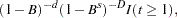CONSTANT

where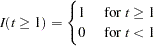length-of-month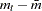where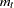= length of month(in days)

(monthly flow)

and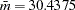(average length of month)

LOM

 stock length-of-month LOMSTOCK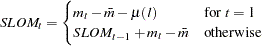where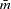andare defined in LOM and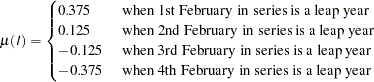length-of-quarter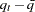where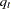= length of quarter(in days)

(quarterly flow)

and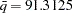(average length of quarter)

LOQ

 leap year (monthly and quarterly flow) LPYEAR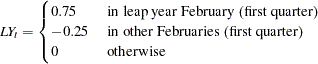fixed seasonal SEASONAL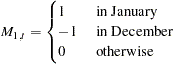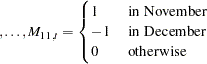fixed seasonal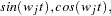SINCOS()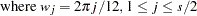andis the seasonal period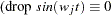for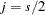)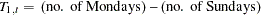TD, TDNOLPYEAR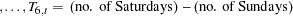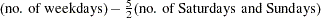TD1COEF, TD1NOLPYEAR

 stock trading day TDSTOCK()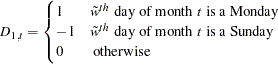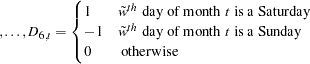where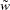is the smaller ofand the length of month.

For end-of-month stock series, setto 31; that is,

specify TDSTOCK(31). Restriction:.

If Easter falls before April, let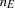be the number of thedays

(monthly or quarterly flow)

on or before Easter that fall in March. Then:

SCEASTER()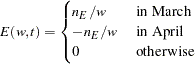If Easter falls on or after April, then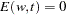.

(Note: This variable isexcept in March and April (or first and

second quarter).) Restriction: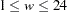.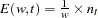and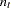is the number of thedays before Easter that fall in month

Easter holiday

(or quarter). (Note: This variable isexcept in February, March,

EASTER()

and April (or first and second quarter).

It is nonzero in February only for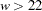.)

Restriction: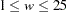.

Labor Day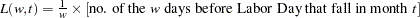LABOR()

(Note: This variable isexcept in August and September.)

Restriction:.

Thanksgiving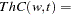proportion of days fromdays before Thanksgiving

THANK()

through December 24 that fall in month(negative values ofindicate

days after Thanksgiving).

(Note: This variable isexcept in November and December.)

Restriction: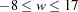.

USERVAR=(variables) < / B=value USERTYPE=option>

specifies variables in the PROC X12 DATA= data set that are to be used as regressors. The variables in the data set should contain the values for each observation that define the regressor; regression variables should also be defined for forecast values if the time series is to be extended with regARIMA forecasts. Missing values are not permitted within the data span, including forecasts, of the user-defined regressors. Example 32.6 shows how you would create an input data set that contains both the series to be seasonally adjusted and a user-defined input variable. Note that all regression variables in the USERVAR= option apply to all time series to be seasonally adjusted unless the MDLINFOIN= data set specifies different regression information.

B=(value <F> ...)

specifies initial or fixed values for the regression parameters in the order in which they appear in the PREDEFINED= and USERVAR= options. Each B= list applies to the PREDEFINED= or USERVAR= variable list that immediately precedes the slash. The PREDEFINED= option and the USERVAR= option cannot be specified in the same REGRESSION statement; however, multiple REGRESSION statements can be specified.

For example, the following statements set an initial value for the user-defined regressor, x, of 1:

```   regression predefined=LOM ;
regression uservar=x / b=1 2 ;
```

In this example, the B= option applies only to the USERVAR= statement. The value 2 is discarded since there is only one variable in the USERVAR= list. To assign an initial value of 1 to the LOM regressor and 2 to the x regressor, use the following statements:

```   regression predefined=LOM / b=1;
regression uservar=x / b=2 ;
```

An F immediately following the numerical value indicates that this is not an initial value, but a fixed value. See Example 32.8 for an example that uses fixed parameters. In PROC X12, individual parameters can be fixed while other parameters in the same model are estimated.

USERTYPE=CONSTANT
USERTYPE=SEASONAL
USERTYPE=TD
USERTYPE=LOM
USERTYPE=LOQ
USERTYPE=LPYEAR
USERTYPE=TDSTOCK
USERTYPE=LOMSTOCK
USERTYPE=EASTER
USERTYPE=LABOR
USERTYPE=THANKS
USERTYPE=AO
USERTYPE=LS
USERTYPE=RP
USERTYPE=HOLIDAY
USERTYPE=SCEASTER
USERTYPE=USER
USERTYPE=TC

enables a user-defined variable to be processed in the same manner as a U.S. Census predefined variable. For instance, the U.S. Census Bureau EASTER() regression effects are included the "RegARIMA Holiday Component" table (A7). You should specify USERTYPE=EASTER to include a user-defined variable which would be processed exactly as the U.S. Census predefined EASTER() variable, including inclusion in the A7 table. Each USERTYPE= list applies to the USERVAR= variable list that immediately precedes the slash. USERTYPE= does not apply to U.S. Census predefined variables. The same rules for assigning B= values to regression variables apply for USERTYPE= options. See the example in B=(value <F> ...).Previous Page | Next Page | Top of Page